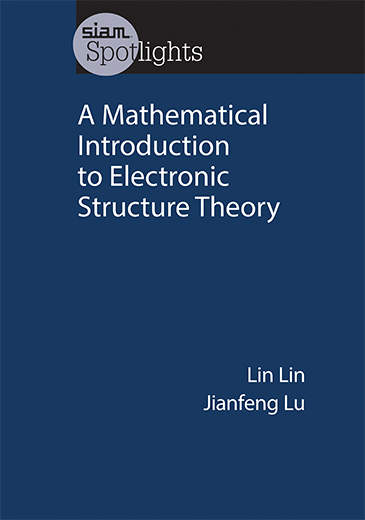This book provides a self-contained, mathematically oriented introduction to the subject and its associated algorithms and analysis. It can help applied mathematics students and researchers with minimal background in physics understand the basics of electronic structure theory and prepare them to conduct research in this area.

You can either download individual chapters of the book (often available with institution access) or purchase from the SIAM book store.Contents

I. Basic theory of quantum mechanics
1. Stern-Gerlach experiment
2. Schrödinger equation in the real space
3. Hydrogen atom
4. Periodic systems
5. Tensor product spaces: two spin-1/2 particles
6. Identical Particles
II. Density functional theory: Formulation and algorithms
1. Hartree-Fock theory
2. Kohn-Sham density functional theory
3. Nonlinear eigenvalue problem
4. Self-consistent field iteration
5. Density matrix formulation
6. Extension to finite temperature
7. Density matrix algorithms
8. Brillouin zone sampling for periodic systems
9. Localization
10. Geometry optimization and ab initio molecular dynamics
11. Time-dependent density functional theory
III. Linear response theory
1. Perturbation of Green’s function
2. Perturbation of the density matrix
3. Density functional perturbation theory
4. Applications of density functional perturbation theory
5. Exponential decay of the Green’s function
6. Time-dependent density functional perturbation theory
7. Perturbation of many-body Hamiltonian
8. Casida formalism
9. Random phase approximation